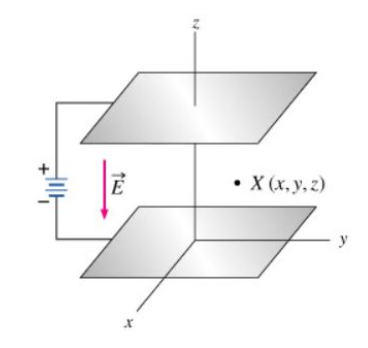# Problem: What is the distance Δz between two surfaces separated by a potential difference ΔV?

###### FREE Expert Solution

The potential at a distance z in a uniform electric field is given by:

V = E•z

When z = 0, V1 = E(0) = 0

80% (142 ratings)###### Problem Details

What is the distance Δz between two surfaces separated by a potential difference ΔV?